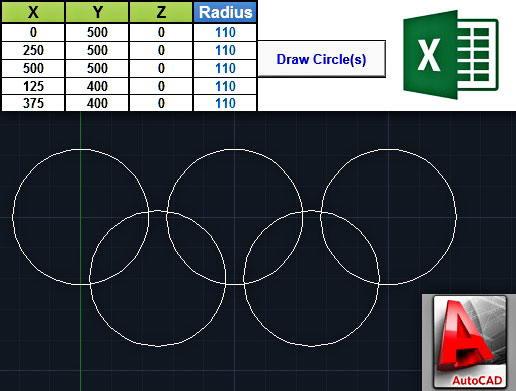# Drawing Circles In AutoCAD Using Excel & VBA

March 5, 2014### Introduction

The idea of drawing objects in AutoCAD by using Excel data has been analyzed several times in this blog. We have seen how to draw polylines, 3D polylines, so, today we will learn how to draw circles. To tell you the truth, the code below was developed as a response to the various requests that I have received the last few weeks from blog readers, as well as from Youtube users.

The code is based on the AddCircle method. According to AutoCAD VBA help, the structure of this method is the following:

Where:
RetVal: Circle object. The newly created Circle object.
Object: ModelSpace Collection, PaperSpace Collection, Block – the objects this method applies to.
Center: Variant (three-element array of doubles); input-only. The 3D WCS coordinates specifying the circle’s center.
Radius: Double; input-only. The radius of the circle. Must be a positive number.
Remarks: This circle is created on the XY plane of the WCS.

The sample workbook that you will find in the Downloads section below requires two main user inputs: the coordinates of the circle center (in X, Y, Z) and the circle radius. Then, by clicking the “Draw Circle(s)” button the circles are being drawn either in the active drawing (if AutoCAD is already lunched), or in a new drawing. If you run the workbook with the sample data, 5 circles will be drawn in AutoCAD, which will look like the Olympic Games logo (a small tribute to the Winter Olympics that finished the previous month).

### VBA code to draw circles in AutoCAD from Excel

The code is actually a loop; almost half of it is used for initializing the AutoCAD object, as well as the active/new drawing.

``````Option Explicit

Sub DrawCircles()

'--------------------------------------------------------------------------------------------------
'Draws circles in AutoCAD using data - circle center coordinates and circle radius - from Excel.
'The code uses late binding, so no reference to external AutoCAD (type) library is required.
'It goes without saying that AutoCAD must be installed at your computer before running this code.

'Written by:    Christos Samaras
'Date:          04/03/2014
'e-mail:        [email protected]
'site:          http://www.myengineeringworld.net
'--------------------------------------------------------------------------------------------------

'Declaring the necessary variables.
Dim LastRow                 As Long
Dim i                       As Long
Dim CircleCenter(0 To 2)    As Double

'Activate the coordinates sheet and find the last row.
With Sheets("Coordinates")
.Activate
LastRow = .Cells(.Rows.Count, "A").End(xlUp).Row
End With

'Check if there are coordinates for at least one circle.
If LastRow < 2 Then
MsgBox "There are no coordinates to draw a circle!", vbCritical, "Circle Center Error"
Exit Sub
End If

'Check if AutoCAD application is open.
On Error Resume Next

'If AutoCAD is not opened create a new instance and make it visible.
End If

'Check (again) if there is an AutoCAD object.
Exit Sub
End If
On Error GoTo 0

'If there is no active drawing create a new one.
On Error Resume Next
End If
On Error GoTo 0

'Check if the active space is paper space and change it to model space.
If acadDoc.ActiveSpace = 0 Then '0 = acPaperSpace in early binding
acadDoc.ActiveSpace = 1 '1 = acModelSpace in early binding
End If

'Loop through all the coordinates/radius and draw the corresponding circle(s).
With Sheets("Coordinates")
For i = 2 To LastRow
'If the circle radius is greater than 0, get the circle center and draw the circle.
'Set the circle centert.
CircleCenter(0) = .Range("A" & i).Value
CircleCenter(1) = .Range("B" & i).Value
CircleCenter(2) = .Range("C" & i).Value
'Draw the circle.
End If
Next i
End With

'Zoom in to the drawing area.

'Release the objects.

'Inform the user about the process.
MsgBox "The circle(s) was/were successfully drawn in AutoCAD!", vbInformation, "Finished"

End Sub ``````

Note that if you have AutoCAD 2010 or a newer version, you will have to download and install the VBA module, otherwise the code will probably fail.

All links were copied from Autodesk‘s website.

### Demonstration video

The short video below demonstrates the result of the above VBA code; 5 circles are drawn in a new AutoCAD drawing based on sample data from the Excel workbook.

The file can be opened with Excel 2007 or newer. Please enable macros before using it.